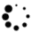() : In animals with bilateral symmetry, the plane of section that is parallel to the longitudinal axis (Barclay, 1803) and passes medial (Schulze, 1893)-lateral (Barclay, 1803), orthogonal to the other longitudinal plane, the sagittal plane (Henle, 1855); divides the body into dorsal (Barclay, 1803) and ventral (Schulze, 1893) parts. The term was introduced by Henle (1855, p. 1); also see Kuhlenbeck (1973, p. 114), Brusca & Brusca (1990, Fig. 4-A), Romer (1962, p. 7), Sandring (2008, p. xxii). In quadrupeds it is often confusingly synonymous with transverse plane (Henle, 1855)-see frontal plane (Horsley & Clarke, 1908)-but it has long been argued that positional terms referring to the horizon or other external landmarks, rather than to internal landmarks, be avoided, especially in comparative anatomy; see Wilder & Gage (1882, pp. 21-23).

() : Synonym for transverse plane (Henle, 1855); introduced by Henle (1855, p. 1), see Standring (2008, Fig. 1). It is often confusingly used in quadrupeds for frontal plane (Henle, 1855); see horizontal plane (Horsley & Clarke, 1908).

() : A plane of section passing through the longitudinal axis (Barclay, 1803) in animals with radial symmetry, dividing the body into roughly equal longitudinal halves; term alluded to by Henle (1855, p. 1). In animals with bilateral symmetry there are two orthogonal longitudinal planes, the sagittal plane (Henle, 1855) and frontal plane (Henle, 1855). A synonym is rostrocaudal plane.

() : The sagittal plane (Henle, 1855) dividing a body into roughly equal right and left halves; term introduced by Henle (1855, p. 1); also see Standring (2008, Fig. 1). Synonyms include midline and midsagittal plane.

() : In animals with bilateral symmetry, the plane of section parallel to the longitudinal axis (Barclay, 1803) of the body and passing dorsal (Barclay, 1803)-ventral (Schulze, 1893), orthogonal to the other longitudinal plane (Henle, 1855), the frontal plane (Henle, 1855). Term introduced by Henle (1855, p. 2), also see Kuhlenbeck (1973, p. 114).

() : The axis orthogonal to the longitudinal axis (Barclay, 1803) or rostrocaudal axis of the body in all animals with a nervous system (Monro, 1783); term introduced by Henle (1855, p. 1). In animals with radial symmetry it is the only axis orthogonal to the longitudinal axis (Barclay, 1803); in animals with bilateral symmetry it is divided into a dorsoventral axis and an orthogonal mediolateral axis with right and left halves; see Kuhlenbeck (1973, p. 112).

() : The plane of section orthogonal to the longitudinal axis (Barclay, 1803), dividing the body into rostral (Schulze, 1893) and caudal (Cleland, 1879) parts. Term introduced by Henle (1855, p. 1), also see Kuhlenbeck (1973, p. 114).